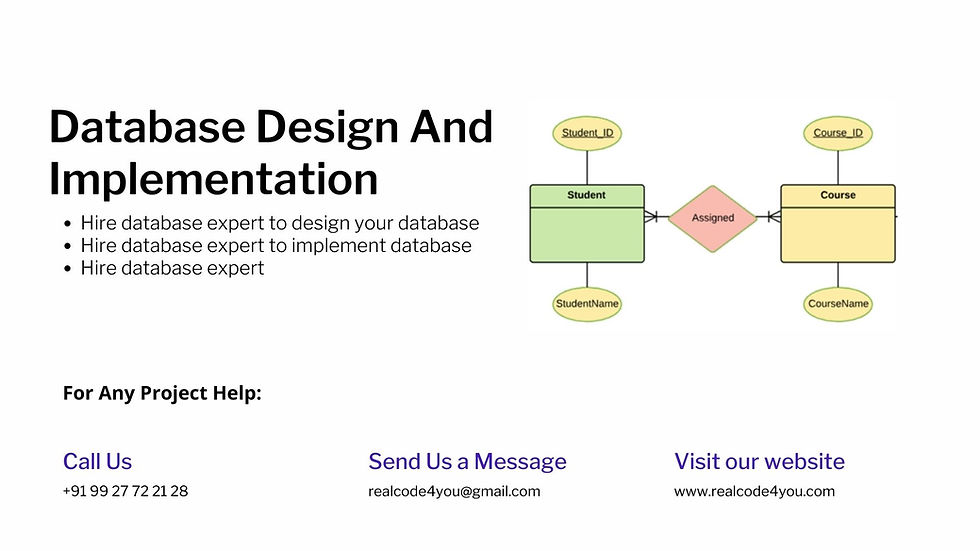top of page
Search

# Database Design And Implementation Help Using SQL Server### Project Requirement

In this you can use company database of any other from your choice.

This task is divided into 3 parts:

• Data Modelling Diagram(s)

• Entity Relationship Diagrams

• Semantic Object Diagram

• Relational Tables

• Normalization tables

• Data Flow Diagram(s) (including context level, level-0 and level-1)

• Data Dictionary

• Use Case Diagram(s) and/ Activity Diagram(s)

• Class Diagram(s)

Necessary Requirements:

• Entity Relationship Diagrams provided and drawn correctly

• Entity Relationship Diagrams were consistent with proposed solution

• Normalize tables into 1 NF

• Normalize tables into 2 NF

• Normalize tables into 3 NF

• Relational Table Diagram Provided and drawn correctly

• Relational Table Diagram corresponds with ERD

• Semantic Object Diagram(s) were provided and drawn correctly

• Semantic Object Diagram(s) are consistent with proposed solution

• Data Flow Diagram(s) (including context level, level-o and level-1) were provided and drawn correctly

• Data Flow Diagram(s) were consistent with proposed solution

• Data Dictionary was provided and drawn correctly

• Data Dictionary was consistent with proposed solution

• Use Case Diagram(s) provided and drawn correctly

• Use Case Diagram(s) were consistent with proposed solution

• Activity Diagram(s) were provided and drawn correctly

• Activity Diagram(s) were consistent with proposed solution

• Class Diagram(s) were provided and drawn correctly

• Class Diagram(s) were consistent with proposed solution

• Create databases

• Create tables

• Apply constraints on the columns

• Create indexes

• Create relationships

• Create views

• Query data using a view

You need follow:

• Create tables

• Assign appropriate data types

• List of validations (8) to be applied on tables

• Apply 8 validations

• Create relationships

• Apply relationship constraints

• Insert records into the tables (At least 5 records in each table)

• List of views to be created

• Create 6 views

• Create SQL query(s) to insert records

• Create SQL query(s) to update records

• Create SQL query(s) to delete records

• Create SQL query(s) using wildcards

• Create SQL query(s) using criterion involving a text field

• Create SQL query(s) using criterion involving a numeric field

• Create SQL query(s) using compound criterion (AND, OR, NOT)

• Create SQL query(s) using multiple functions in the same command

• Create SQL query(s) to group records

• Create SQL query(s) to Sort results

• Create SQL query(s) to join tables

• Write functions ,

• procedures,

• triggers

What is necessary:

• Explanation of at least 4 stored procedures including insert , update , delete and select queries

• Use of input parameters in stored procedures

• Use of output parameters in stored procedures

• Use of default values in stored procedure

• Execute code for stored procedures

• Create reports based on functions created above

• Create reports based on stored procedure created above

• Create a report that demonstrates parent-child relationship

• Create a report that demonstrates group by clause

• Format reports appropriately

• Create at least 1 trigger